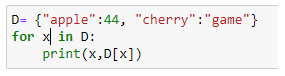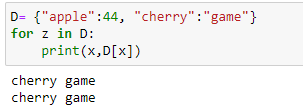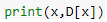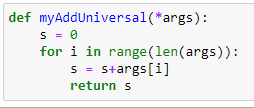# Control flow dictionaries

Hi friends, I am taking the 12 hour course of Python for Data Science of FreeCodeCamp in Youtube (Python for Data Science - Course for Beginners (Learn Python, Pandas, NumPy, Matplotlib) - YouTube) and I have a doubt:

I have the next syntaxis of code:Which gives this result:

apple 44
cherry game

And what happens is that I don’t understand what the code refers to “for x in D”. I understand that D refers to the dictionary, but what does “for x” refer to? What is x in that dictionary? I tried to change “for x” to “for z” and it gives me the following result:

cherry game
cherry game

So what do those variables refer to in that dictionary?

Also why the professor types print(x, D) instead of just print(x)?

Thanks.

`x` is just a variable name that represents the Dictionary keys that are iterated over. You could name the variable anything. Typically, you should name it what the variable represents. I would name the variable `key` because that is what it is.

You will need to post your actual code to show us how you produced the above output, because only changing the variable to `z` would not do this.

Thanks Randel,

This is the code with “for z”:There must be code above the 1st line shown because if that is the only code, then it would error out as `x` is not defined anywhere

I assume the following line is above the code you posted:

``````x = "cherry"
``````

No, that’s the only code I have. It’s a new notebook in Jupyter.

Also, I would like to ask you why do the professor typesinstead of just print(x).

Thanks.

Printing just `x` would just print the Dictionary keys like:

``````"apple"
"cherry"
``````

I would have to see the notebook.

Like I said, there had to be some code above the other code you posted. In the first `for in` loop, the last value of `x` is “cherry” which is why you get:

``````cherry game
cherry game
``````

printed. The second `for in` loop just prints `x` and `D[x]` twice.

I have another question.

In this code, what is the value of “i” and “s”. I didn’t define them before, so what are they?Thanks.

The `s = 0` is defining `s` and initializing its value to `0`.

The `i` gets defined when it is written in the `for in range`.

They are just variables. I assume the `s` starts a zero so the line:

``````s = s + args[i]
``````

can work. In the end, it just represents a sum, though the return statement is not indented correctly to achieve the sum of all the arguments passed to the function.

So the value of `S` is zero, but what is the value of `i` after` for in range` is coded? What is the range there? Between which numbers?

Thanks.

You should probably take a beginners course in Python before trying to learn Python for Data Science. Do some research on the `range` method and you learn what the first value of `i` is in a `for i in range` loop.